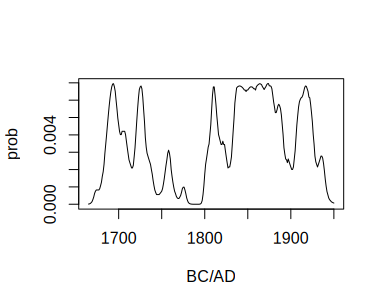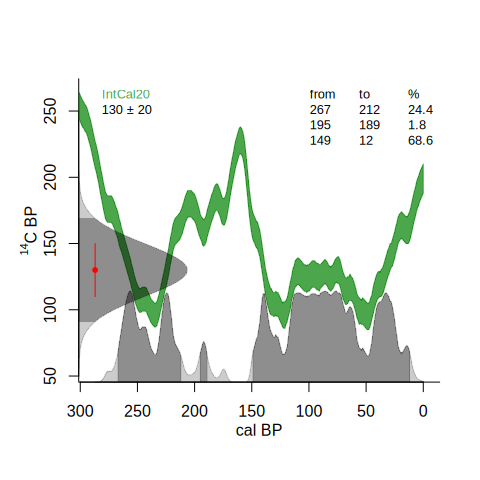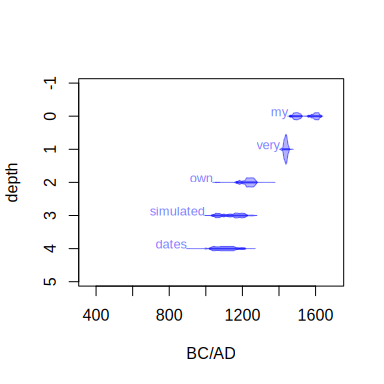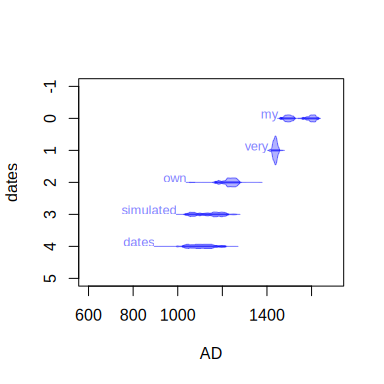3. calculations

The IntCal package also provides some functions related to radiocarbon calibration. First there are two functions to calculate radiocarbon ages from pMC values (in this case of a postbomb date):

pMC.age(150, 1)
##  -3257    53

and the other way round:

age.pMC(-2300, 40)
##  133.000   0.661

We can also obtain the calibrated probability distributions from radiocarbon dates, e.g. one of 130 +- 20 C14 BP:

plot(calib.130, type="l")For reporting purposes, calibrated dates are often reduced to their 95% highest posterior density (hpd) ranges (please report all, not just your favourite one!):

hpd(calib.130)
##      from   to perc
## [1,] 1683 1738 24.4
## [2,] 1755 1761  1.8
## [3,] 1801 1938 68.6

Want a plot of the radiocarbon and calibrated dates, together with their hpd ranges?

calibrate(130,20)You can also draw one or more calibrated distributions:

set.seed(123)
dates <- sort(runif(5, 100, 1000))
errors <- .05*dates
depths <- 0:4
labels <- c("my", "very", "own", "simulated", "dates")
draw.dates(dates, errors, depths, BCAD=TRUE, labels=labels, cal.lim=range(dates, 600, 1700))or add them to an existing plot:

plot(0, type="n", xlim=c(600, 1700), ylim=c(5,-1), xlab="AD", ylab="dates")